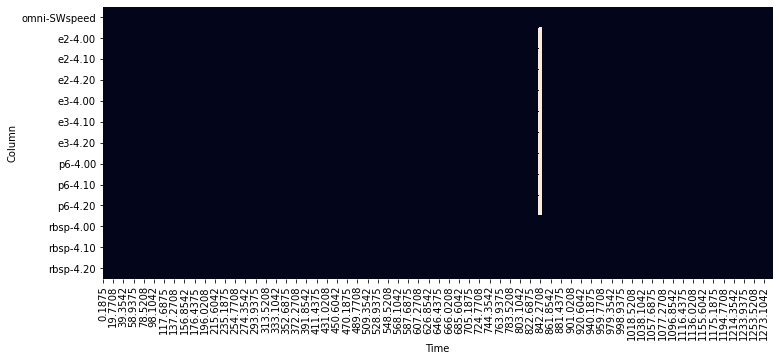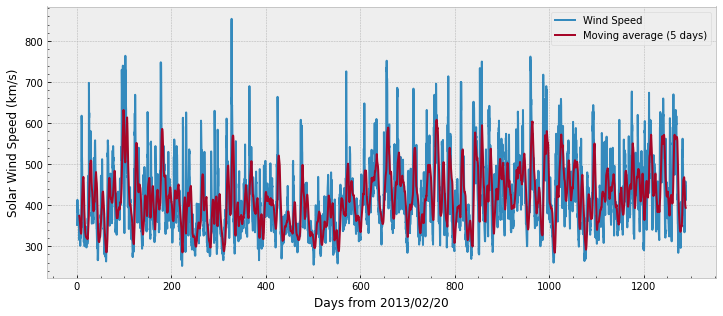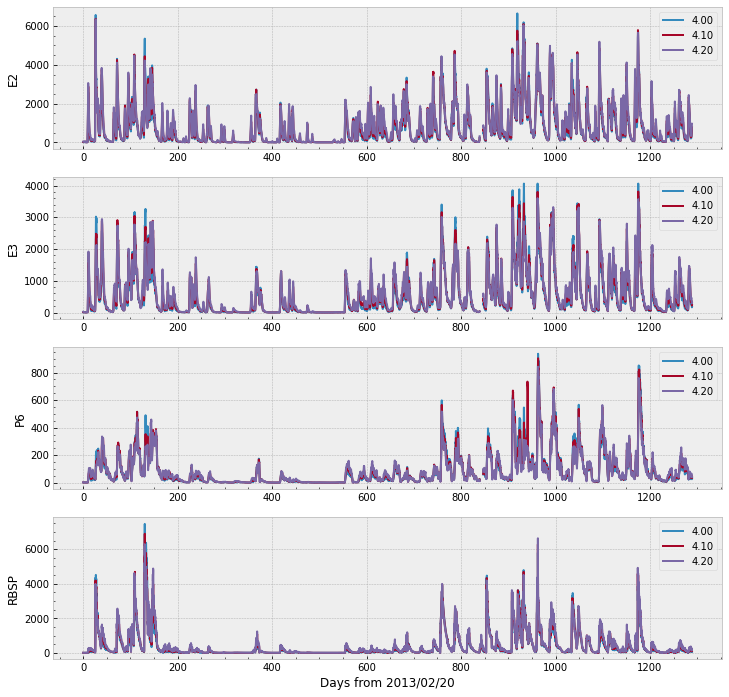# Notebook¶

Man‐made satellites operating in medium‐ and high‐altitude Earth orbits are continuously exposed to hazardous space radiation originated from different sources. A major contributor to such hazardous environment are the relativistic electron population trapped within Earth’s outer Van Allen belt. Electrons are deemed relativistic when they contain energies comparable to and/or larger than their rest energy of 0.511 megaelectron‐volt (MeV). Due to their high penetration capability, these MeV electrons are difficult to be fully stopped by normal shielding. Given that the successful NASA Van Allen Probes mission, also known as RBSP (Mauk et al., 2013), ended in October 2019, the need of reliable forecasting models for MeV electrons becomes compelling once again due to the absence of in situ measurements.

In this notebook, we use a machine learning approach to forecast the fluxes of one MeV electrons in Earth’s outer radiation belt using as input measurements of precipitating electrons together with upstream solar wind speeds. We use linear regression from the Scikit-Learn, as well as a model created with TensorFlow.

The analysis that follows is a small subset of the work published in Pires de Lima et al. (2020): Forecasting Megaelectron‐Volt Electrons Inside Earth’s Outer Radiation Belt: PreMevE 2.0 Based on Supervised Machine Learning Algorithms. If you use any of this code, we ask that you cite Pires de Lima et al. (2020).

from IPython.display import YouTubeVideo


To perform the forecasting analysis, we’ll look at data from three main sources:

• OMNI

• This data set includes the geomagnetic disturbance index Dst and upstream solar wind conditions.

• POES

• These are measurements from low-Earth-orbit satellite POES-15 for electrons with different energies.

• RBSP

• RBSP measurements are the target of the model–the 1 MeV electrons.

The OMNI data contains solar wind magnetic field and plasma measurements from spacecraft orbiting about the L1 Lagrange point $$\sim$$225 Re in front of the Earth. POES data contains the spatial distributions of >100 keV, >300 keV and >1000 kev electrons observed in low Earth orbit. RBSP data contains the radial distributions of 1MeV electrons observed by RBSP-a.

The data we will use for forecasting were prepared by Yue Chen at LANL and cover the time between February 20, 2013 and August 31, 2016.

The next cell imports the necessary files for the analysis:

import os                       # manage filepaths
from zipfile import ZipFile     # unzips the data

with open('example.zip', 'wb') as f:
f.write(r.content)

with ZipFile('example.zip', 'r') as zfile:
zfile.extractall()

# rename the folder to 'data'
os.rename('example', 'data')

for f in os.listdir('data'):
print(f)

Input_data_POES_E3_300keV_electrons_20130220-20160831_example.txt
Input_data_POES_E2_100keV_electrons_20130220-20160831_example.txt
Input_data_OMNI_20130220-20160831.txt
Target_data_RBSP_1MeV_electrons_20130220-20160831_example.txt
Input_data_POES_P6_1000keV_electrons_20130220-20160831_example.txt


The cell above downloaded the files we need and extraced the files into a folder called example. Then, we listed the files in the example folder. The file naming convention helps us quickly see what are the targets and inputs, as well as the source of the data and the time span of the data present.

The next cell imports all the remaining libraries we’ll use in this notebook:

import numpy as np     # manage with n-dimensional arrays
import pandas as pd    # manage tabular data

from matplotlib import pyplot as plt    # for most plots
import seaborn as sns                   # easier setup for some types of plots
import plotly.express as px             # for interactive plots

# a workaround to show plotly in jupyter books
from IPython.core.display import display, HTML
from plotly.offline import init_notebook_mode, plot
init_notebook_mode(connected=True)

from sklearn.metrics import r2_score               # to measure models' performances
from sklearn.preprocessing import StandardScaler   # to scale data
from sklearn.linear_model import LinearRegression  # OLS linear model
import tensorflow as tf                            # CNN model

from timeit import default_timer as timer   # to measure this notebook execution time

start_time = timer()

/usr/local/lib/python3.6/dist-packages/statsmodels/tools/_testing.py:19: FutureWarning: pandas.util.testing is deprecated. Use the functions in the public API at pandas.testing instead.
import pandas.util.testing as tm


Now that we downloaded the data and imported the necessary libraries, we can continue with the analysis.

## 1. Data Input and Visualization¶

The data was pre-processed and is now corrected and binned into five hours windows. Before we start with the analysis, let’s check the files.
Data from OMNI:

with open("data/Input_data_OMNI_20130220-20160831.txt") as f:
for _ in range(15):
print(next(f), end='')

;Produced by Yue Chen, @LANL, on Jul  3, 2018
;
;Data used as inputs for forecasting models: OMNI data between 20130220 and 20160831
;Columns: S/N, Time (days in hr, counted from 20130220 00:00:00), Dst(nT), upstream solar wind speed (km/s), upstream solar wind proton density
;

;format (I5, 3x, f15.4, 3e15.2)

S/N              Time            Dst      S/W speed     S/W p+ den
1            0.1875      -3.50e+00       3.52e+02       9.00e+00
2            0.3958      -3.50e+00       3.86e+02       6.95e+00
3            0.6042      -1.85e+01       3.90e+02       6.00e+00
4            0.8125      -1.30e+01       3.84e+02       6.60e+00
5            1.0208      -1.20e+01       4.13e+02       9.20e+00


The head of the OMNI file shows that the file contains a header explaining the data that is organized in columns.
Let’s check the RBSP target data format:

with open("data/Target_data_RBSP_1MeV_electrons_20130220-20160831_example.txt") as f:
for _ in range(15):
print(next(f), end='')

;Produced by Yue Chen, @LANL, on Jul  3, 2018 - Lshell selection performed by Rafael Pires de Lima, on August 12, 2020
;
;Data to be compared to model forecast results: Radial distributions of 1MeV electrons observed by RBSP-a between 20130220 and 20160831
;Columns: S/N, Time (days in hr, counted from 20130220 00:00:00), e- fluxes at L1/L2, .../Ln
;L values are shown in the top row

;format (I5, 3x, f10.4, 43e10.2)

S/N         Time       4.00      4.10      4.20
1       0.1875   1.78e+00  2.59e+00  4.96e+00
2       0.3958   1.25e+00  1.95e+00  3.51e+00
3       0.6042   8.84e-01  1.46e+00  2.49e+00
4       0.8125   1.15e+00  1.83e+00  3.38e+00
5       1.0208   1.17e+00  1.91e+00  3.47e+00


Much like the OMNI data, the RBSP data contains a header and the data is organized in columns. However, unlike the OMNI data that contains multiple information, the RBSP has only fluxes at different L-shells. Here, we see only L-shells 4.00, 4.10, and 4.20. The POES input has the same format, so we will not display the head of the files here.

To make file managing easier, we define a dictionary in the next cell:

data_dir = './data/'
dict_files = {'omni': os.path.join(data_dir, 'Input_data_OMNI_20130220-20160831.txt'),
'e2':   os.path.join(data_dir, 'Input_data_POES_E2_100keV_electrons_20130220-20160831_example.txt'),
'e3':   os.path.join(data_dir, 'Input_data_POES_E3_300keV_electrons_20130220-20160831_example.txt'),
'p6':   os.path.join(data_dir, 'Input_data_POES_P6_1000keV_electrons_20130220-20160831_example.txt'),
'rbsp': os.path.join(data_dir, 'Target_data_RBSP_1MeV_electrons_20130220-20160831_example.txt'),
}


Now it is much easier to access the files if we need to. For example, the address to the target data file:

print(dict_files['rbsp'])

./data/Target_data_RBSP_1MeV_electrons_20130220-20160831_example.txt


The fact that the files have been preprocessed help in the analysis as they have similar format, but we still need to read the data into Python. Pandas is a very helpful library to work with data organized in columns, so we’ll use it to manage the data. Pandas main object for two-dimensional tabular data is called a dataframe.

We could read file by file into independent dataframes with something like:

# read rbsp data


But we will make use of the dictionary created before to help manage the files and read all the data using a single loop:

dfs = {}
for name in dict_files:
if name == 'omni':
# there are spaces in the omni columns names:
skiprows=10,
sep='\s+',
names=['S/N', 'Time', 'Dst', 'SWspeed', 'SWpden'])
dfs[name] = dfs[name].set_index(['S/N', 'Time'])
# the only column we keep is the solar wind speed:
dfs[name] = dfs[name]['SWspeed']
else:
skiprows=9,
sep='\s+',
index_col=['S/N', 'Time'])


We can then concatenate all the dataframes stored in the dictionaries into a single dataframe with a MultiIndex:

df = pd.concat(dfs, axis=1)
df

omni e2 e3 p6 rbsp
SWspeed 4.00 4.10 4.20 4.00 4.10 4.20 4.00 4.10 4.20 4.00 4.10 4.20
S/N Time
1 0.1875 352.0 11.50 20.2 41.7 6.45 12.1 25.9 2.24 2.72 4.13 1.780 2.59 4.96
2 0.3958 386.0 9.11 16.1 34.0 5.53 10.4 21.7 1.96 2.32 3.32 1.250 1.95 3.51
3 0.6042 390.0 8.70 15.2 30.6 5.67 10.1 20.1 1.85 2.25 3.01 0.884 1.46 2.49
4 0.8125 384.0 8.64 14.3 28.2 5.95 10.1 19.3 1.80 2.23 2.90 1.150 1.83 3.38
5 1.0208 413.0 8.59 14.5 28.5 6.00 10.3 19.6 1.81 2.14 2.72 1.170 1.91 3.47
... ... ... ... ... ... ... ... ... ... ... ... ... ... ...
6183 1288.1042 426.0 367.00 482.0 597.0 250.00 329.0 410.0 38.90 48.80 57.70 92.200 150.00 209.00
6184 1288.3125 458.0 431.00 570.0 713.0 230.00 305.0 381.0 36.40 45.80 54.20 113.000 159.00 218.00
6185 1288.5208 425.0 485.00 643.0 797.0 187.00 250.0 316.0 29.50 36.20 43.20 92.400 138.00 206.00
6186 1288.7292 412.0 538.00 710.0 882.0 164.00 222.0 288.0 26.20 33.30 40.20 113.000 180.00 247.00
6187 1288.9375 426.0 596.00 778.0 959.0 175.00 237.0 306.0 27.50 36.00 42.20 66.200 113.00 154.00

6187 rows × 13 columns

The output from the cell above shows the dataframe df organization. S/N and Time are the row indexes. S/N and Time are, respectively, an integer marker and days in hours, counted from 00:00:00 February 20, 2013. The following columns contain the actual data we use in the analysis: omni, e2, e3, p6, and rbsp:

• omni: solar wind speed in km/s

• e2: < 100 keV electron fluxes

• e3: < 300 keV electron fluxes

• p6: < 1000 keV electron fluxes

• rbsp: 1MeV electron fluxes

As S/N and Time represent the same thing, we drop one of the levels:

df = df.droplevel('S/N')


Now is a good opportunity to explore the data. We first start making a plot to see if there are any missing values:

fig, ax = plt.subplots(1, figsize=(12,5))
sns.heatmap(df.isnull().T, cbar=False, ax=ax)
ax.set_ylabel('Column');The figure depicting the null values in the dataframe (df.isnull()) above shows a white line around Time $$\sim 840$$. That is an indication that there are no measurements in that period.

We can also plot the data for visualization:

# plot OMNI with specific aesthetics:
with plt.style.context('bmh'):
fig, ax = plt.subplots(1, figsize=(12,5))
ax.plot(df.index.get_level_values('Time'), df['omni'],
label='Wind Speed')
ax.plot(df.index.get_level_values('Time'), df['omni'].rolling(24).mean(),
label='Moving average (5 days)')
ax.set_ylabel('Solar Wind Speed (km/s)')
ax.set_xlabel('Days from 2013/02/20')
ax.legend()
ax.minorticks_on()We can plot the remaining of the data in a single figure with the code below. Note that the range goes over 6,000 for E2, E3, and RBSP (the target), whereas P6 does not cross 1,000.

with plt.style.context('bmh'):
fig, ax = plt.subplots(nrows=4, figsize=(12,12))
for ii, source in enumerate(['e2', 'e3', 'p6', 'rbsp']):
df[source].plot(ax=ax[ii]) # Pandas plot function
ax[ii].minorticks_on()
ax[ii].set_ylabel(source.upper())
ax[ii].set_xlabel('')
ax[-1].set_xlabel('Days from 2013/02/20')It would be interesting to have some interactivity and to see all the data in a single plot. One possible approach is to melt the dataframe to a long format and use Plotly.

Let’s first melt the dataframe, discarding the OMNI data:

melted_df = df.drop('omni', axis=1).reset_index().melt(id_vars='Time', var_name = ['source', 'L-shell'])
melted_df

Time source L-shell value
0 0.1875 e2 4.00 11.50
1 0.3958 e2 4.00 9.11
2 0.6042 e2 4.00 8.70
3 0.8125 e2 4.00 8.64
4 1.0208 e2 4.00 8.59
... ... ... ... ...
74239 1288.1042 rbsp 4.20 209.00
74240 1288.3125 rbsp 4.20 218.00
74241 1288.5208 rbsp 4.20 206.00
74242 1288.7292 rbsp 4.20 247.00
74243 1288.9375 rbsp 4.20 154.00

74244 rows × 4 columns

The next cell creates an interactive plot. You can zoom and pan to see the data. You can also select the data by clicking on the legend on the right. Although Plotly is currently able to render directly int jupyter notebooks, the figure may not render properly if we use fig.show in jupyter-books. The workaround to render Plotly in a jupyter-book is to save the file and use iPython’s display.

fig = px.line(melted_df, x='Time', y='value', color='source', animation_frame='L-shell')
# the next line should work in most notebooks
# fig.show()
# but we use the workaround to render the figure
plot(fig, filename=os.path.join(data_dir, 'figure1_data.html'))

'./data/figure1_data.html'

display(HTML(os.path.join(data_dir, 'figure1_data.html')))